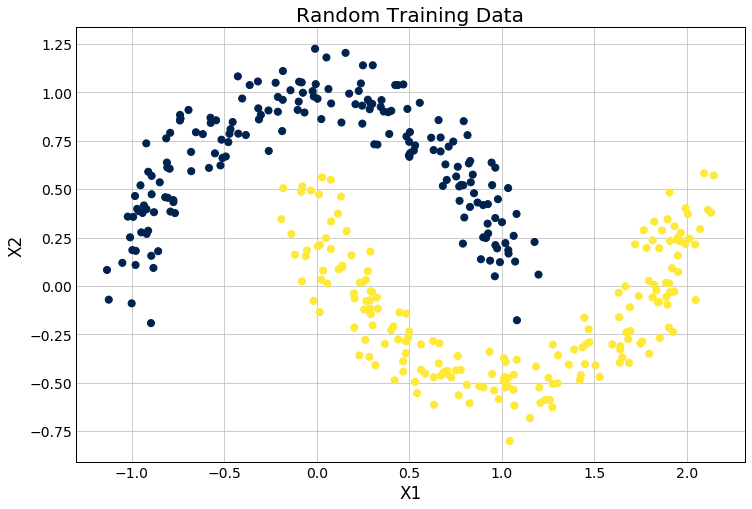# Deep Learning 101 — Building a Neural Network from the Ground Up — DataHubbs

Source: Deep Learning on Medium# Deep Learning 101 — Building a Neural Network from the Ground Up — DataHubbs

In the last post, we walked through the theory behind deep learning and introduced key concepts like backpropagation and gradient descent. Here, we take a more hands-on approach and show how this can all be implemented using Python and Numpy. I’ll show where the theory comes in here as we build a simple neural network architecture for prediction, so familiarity with the concepts discussed previously will be helpful. As I have mentioned previously on this blog, I always find that having some understanding the guts of the algorithm (even if your understanding is not perfect) greatly helps when working in data science and machine learning. You can typically understand where a given algorithm might perform better or worse, trouble shoot better, and grasp the limitations and potential more readily. Additionally, familiarizing yourself with such a low-level implementation is also helpful when you move to other, higher-level frameworks like TensorFlow or Keras to build an understanding of what is going on there.

Note: Medium still doesn’t render mathematical equations correctly, so if you want to see the details, check out my post here.

# TL;DR

We build a neural network from scratch using nothing by Python and the Numpy package. I walk through the architecture step-by-step and explicitly call out the what, why, and how.

# Getting Started

If you’re following along at home, fire up your favorite Python IDE and get going! We’ll build a network line by line from scratch to make a prediction from some fake-data we can generate using scikit-learn and then, build a few helper functions around this to make our predictions.
Without further ado, let’s get to importing our packages.

import numpy as np from sklearn.datasets import make_moons from sklearn.model_selection import train_test_split import matplotlib.pyplot as plt

We’re going to need some data to train on. Thankfully sklearn has a number of different data sets to work with.

np.random.seed(0) X, Y = make_moons(500, noise=0.1) # Split into test and training data X_train, X_test, Y_train, Y_test = train_test_split(  X, Y, test_size=0.25, random_state=73) # Plot results plt.figure(figsize=(12,8)) plt.scatter(X_train[:,0], X_train[:,1], c=Y_train, cmap=plt.cm.cividis, s=50) plt.xlabel('X1') plt.ylabel('X2') plt.title('Random Training Data') plt.show()